# Numerical Investigation with Stability Convergence Analysis of Chemically Hydromagnetic Casson Nanofluid Flow in the Effects of Thermophoresis and Brownian Motion

Numerical Investigation with Stability Convergence Analysis of Chemically Hydromagnetic Casson Nanofluid Flow in the Effects of Thermophoresis and Brownian Motion

Munmun Mondal Rajib BiswasKazi Shanchia Mehedy Hasan Sarder F. Ahmmed

Mathematics Discipline, Khulna University, Khulna-9208, Bangladesh

Corresponding Author Email:
rajibkumath11@gmail.com
Page:
59-70
|
DOI:
https://doi.org/10.18280/ijht.370107
16 March 2018
|
Accepted:
23 July 2018
|
Published:
31 March 2019
| Citation

OPEN ACCESS

Abstract:

In this evaluation, a numerical exploration is inscribed on MHD unsteady heat and mass transfer of Casson nanofluid flow where variable thermal conductivity, radiation and heat absorption are counterfeited. The model is implemented for the nanofluid which annexes the impression of thermophoresis and Brownian motion. The governing models are metamorphosed as a dimensionless silhouette by the renovation rule of mathematics and the procured dimensionless couple of partial differential equations (PDEs) are elucidated by utilizing explicit finite difference method (EFDM). The numerical aggregates are enumerated for the miscellaneous dimensionless parameters on velocity, temperature and concentration profiles along with the skin friction, Nusselt number and Sherwood number with distinct time interims. ForTran programing language is manipulated as the predominated software for executing the numerical values. Also, by wielding the initial boundary conditions U=T=C=0, DX=0.83 and DY=0.50 for Dt=0.0005, convergence criteria are exhibited with Sc³ 0.18 and Le³0.20. At the end, the prevailed culminations are illustrated and perused after the stability convergence test (SCT) by manoeuvring graphics software tecplot-9 and streamlines and isotherms are delineated.

Keywords:

casson nanofluid, EFDM, radiation, chemical reaction, MHD, porous medium

1. Introduction

Casson fluid is a non-Newtonian shear thinning fluid appending with infinite viscosity at zero shear rates. Pragmatic implementations of Casson fluid comprise in the sector of petroleum drilling, polymer engineering, certain separation processes, manufacturing of foods and paper. In addition, biological, chemical, medical and engineering applications are vitally pivotal affiliated to the Casson fluid. Distinct inquisitors have implemented their fact-finding pursuit in this direction enlightening the consequences of numerous parameters on the flow of the Casson fluid. Unsteady Casson nanofluid flow over a stretching sheet have been appraised by Oyelakin et al. . Kataria and Patel  have scrutinized the Soret and heat generation sequels on MHD Casson fluid flow. The repercussions on magnetic field in squeezing flow of a Casson fluid have been probed by Ahmed et al. . Furthermore, Mahanta et al.  perused the 3D Casson fluid flow. In very recent time, Afikuzzaman et al. ; Biswas et al. ; Afikuzzaman et al.  and Wahiduzzaman et al.  have numerically evaluated unsteady MHD Casson fluid flow. Also, Casson nanofluid has been contemplated by abundant scrutinisers such as: Biswas et al. ; Khalid et al. ; Ali et al.  and Ghadikolaei et al. .

A nanofluid is a fluid, mingle with nanometer-sized particles typically made of metals, oxides and carbides. In 1995, the abstraction of nanofluids has been initiated by Chai et al. , where the breach of nanaparticles in a common base fluids include water, ethylene glycol and oil has proffered. Nanofluids have some substantial properties that assemble them potentially convenient in many exertions in heat transfer, including microelectronics, fuel cells, and pharmaceutical processes. Ganga et al.  delineated the MHD flow of Boungiorno model nanofluid over a vertical plate. In addition, optimal interpretation of nonlinear heat and mass transfer in a two-layer flow with nano-Eyring–Powell fluid was assembled by Khan et al. . Denouements of magnetic field and radiation absorption on mixed convective Jeffrey nanofluid flow have been dispatched by Biswas et al. . Explicit numerical study of unsteady hydromagnetic mixed convective nanofluid flow elaborated by Beg et al. . Heat transfer of MHD nanofluid in disparate view have been propounded by multifarious assessors analogous as Dogonchi et al. ; Dogonchi et al. ; Ahmmed et al. ; Leekea et al. ; Uddin et al. ; Bilal et al.  and Arani et al. .

In the past several decades, the evaluation of magneto-hydrodynamic (MHD) mass transfer flow has procured apocalyptic to profuse scholar’s due to its diverse industrial applications such as the cooling of reactors, power generators, electrostatic precipitation, polymer technology petroleum industry, aerodynamics heating, petroleum industry, the operations of MHD accelerators, fluid droplets sprays, agriculture, geophysics, metrology, petro-chemical industry and so on.   Heat and mass transfer sequels on MHD natural convective flow was implemented by Murthy et al. . Das et al.  have instigated magnetohydrodynamic mixed convective slip flow. Convective heat transfer and MHD viscoelastic nanofluid flow has been actuated by Shit et al. . In contemporary epoch, MHD has become a great fact-finding argument of computational fluid dynamics (CFD) which contrived by multitudinous scientists commensurate as Ibrahim ; Rani and Kim ; Animasaun and Oyem  and Lavanya and Ratnam .

Homogeneous to MHD deportment, chemical reaction is a stratagem that assists to the transformation of one set of chemical substances to another at a fixed temperature and concentration. Heat and mass transfer on MHD mixed convection axisymmetric chemically reactive flow of Maxwell fluid flow was evoked by Khan et al. . Also, Tripathy et al.  have illustrated the chemical reaction effect. Here, authors have renovated their governing equations into a two-point boundary value predicament manipulating similarity variables and then elucidated numerically by forth order Runge-Kutta method with shooting modus operandi.

Electromagnetic radiation has anonymous conventional characteristics depending on the frequency and wavelengths of the radiation simulates a vital role in industrial and technological areas such as various space vehicles, satellites, missiles, aircraft, gas turbines and nuclear reactors. Das et al.  perused Hall denouements with radiative heat transfer past a porous plate. They disentangled their governing equations by wielding fourth order Runge-Kutta- Fehlberg method with the shooting technique. Thermal radiation culmination was elaborated by Dogonchi by et al. . In addition, combined electrical MHD heat transfer with radiative and viscous dissipation out-turns have been unveiled by Hsiao ; Biswas et al. ; Ahmmed et al.  and Biswas et al. .

The intention of the contemporaneous interpretation is to evaluate the sequels of MHD unsteady heat and mass transfer of Casson nanofluid flow past an exponentially accelerated plate. The consecutive Casson nanofluid model is reassessed to affix the consequence of thermophoresis and Brownian motion. We have pivoted the culminations of heterogeneous parameters such as of Casson parameter, permeability of porous medium, radiation parameter, Lewis number, thermophoresis parameter, Brownian motion parameter, Soret number, magnetic parameter, Schmidt number, Prandtl number, Heat source parameter, chemical reaction parameter, Grashof number and modified Grashof number. Furthermore, we have evaluated the thermal and momentum boundary layer thickness with isotherms and streamlines analysis. Finally, the present outcomes have been analogized with the foregoing ramifications.

2. Mathematical Analysis

The unsteady two dimensioAccording to Oyelakin et al.  and Biswas et al. , the rheological equation of Casson fluid flow has been contemplated as:nal free convection Casson nanofluid flow of an electrically conducting viscous incompressible fluid through a vertical porous permeable plate in the manifestation of thermal radiation, thermal diffusion and chemical reaction are enumerated with the x-axis is contemplated along the plate in the precipitously up sloping orientation and the y-axis is stipulated normal to the plate. A uniform magnetic field strength B0 is implemented across to the flow direction. It is appraised that the temperature of the wall is Tw and concentration at the plate is Cw at initially which are constant but at time t>0. The plate is precipitated exponentially with a velocity u=U0 exp(a´t) in its own plane and the temperature and concentration level of the plate are elevated exponentially to Tw(=T$\infty$) and Cw(=C$\infty$) respectively with time t. The physical configuration and coordinate system of this present model is unveiled in Figure 1.

## 1.png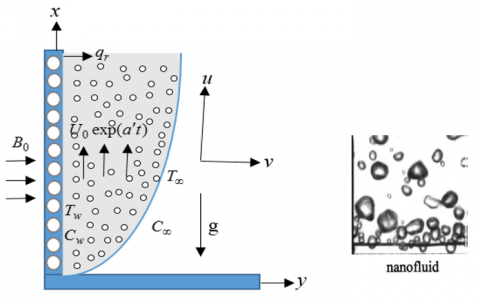Figure 1. Physical configuration

According to Oyelakin et al.  and Biswas et al. , the rheological equation of Casson fluid flow has been contemplated as:

${{\tau }_{ij}}=2\left( {{\mu }_{b}}+\frac{{{P}_{y}}}{\sqrt{2\pi }} \right){{e}_{i\,j}}\,\,\,\,\,\,\text{when}\,\,\pi >{{\pi }_{c}}$ (1)

${{\tau }_{ij}}=2\left( {{\mu }_{b}}+\frac{{{P}_{y}}}{\sqrt{2{{\pi }_{c}}}} \right){{e}_{i\,j}}\,\,\,\,\,\,\text{when}\,\pi <{{\pi }_{c}}$ (2)

where, $\tau$ij is the component of stress tension, π is the product of the component of deformation rate with itself, eij is (i, j)th component of the deformation rate, πc is critical value based on the non-Newtonian model and Py is comprehended as yield stress of the fluid which is verbalized as:

$P_y=(μ_b √2π)/β$

where, $\mu$b = plastic dynamic viscosity of Non-Newtonian fluid. In a case of Casson fluid flow, where $\pi >{{\pi }_{c}}$, therefore

$μ=μ_b+P_y/√2π or, μ=μ_b+((μ_b √2π)/β)/√2π or, υ=μ_b/ρ (1+1/β)$

Under these speculations, the pivotal dimensional governing equations with boundary conditions can be asserted as:

$\frac{\partial u}{\partial x}+\frac{\partial v}{\partial y}=0$ (3)

$\frac{\partial u}{\partial t}+u\frac{\partial u}{\partial x}+v\frac{\partial u}{\partial y}=\upsilon \left( 1+\frac{1}{\beta } \right)\frac{{{\partial }^{2}}u}{\partial {{y}^{2}}}+g{{\beta }_{T}}\left( T-{{T}_{\infty }} \right)+g{{\beta }_{C}}\left( C-{{C}_{\infty }} \right)-\left( 1+\frac{1}{\beta } \right)\frac{\upsilon }{{{k}^{*}}}u-\frac{\sigma B_{0}^{2}u}{\rho }$ (4)

$\frac{\partial T}{\partial t}+u\frac{\partial T}{\partial x}+v\frac{\partial T}{\partial y}=\frac{1}{\rho {{C}_{p}}}\frac{\partial }{\partial y}\left( k\frac{\partial T}{\partial y} \right)-\frac{1}{\rho {{C}_{p}}}\frac{\partial {{q}_{r}}}{\partial y}+{\tau }'\left\{ {{D}_{B}}\left( \frac{\partial C}{\partial y}\frac{\partial T}{\partial y} \right)+\frac{{{D}_{T}}}{{{T}_{\infty }}}{{\left( \frac{\partial T}{\partial y} \right)}^{2}} \right\}+\frac{Q}{\rho {{C}_{p}}}\left( T-{{T}_{\infty }} \right)$ (5)

$\frac{\partial C}{\partial t}+u\frac{\partial C}{\partial x}+v\frac{\partial C}{\partial y}={{D}_{m}}\frac{{{\partial }^{2}}C}{\partial {{y}^{2}}}-{{K}_{1}}(C-{{C}_{\infty }})+{{D}_{B}}\frac{{{\partial }^{2}}C}{\partial {{y}^{2}}}+\frac{{{D}_{T}}}{{{T}_{\infty }}}\frac{{{\partial }^{2}}T}{\partial {{y}^{2}}}+\frac{{{D}_{m}}{{K}_{T}}}{{{T}_{m}}}\frac{{{\partial }^{2}}T}{\partial {{y}^{2}}}$ (6)

The corresponding boundary conditions are:

\left. \begin{align} & t\le 0,\,u=0,\,v=0,\,\,T={{T}_{w}},\,C={{C}_{w}}\,\,\,\,\,\,\,\,\,\text{for}\,\,\text{all}\,\,y \\ & t>0,\,u={{U}_{0}}\,{{e}^{\left( {a}'t \right)}},\,v=0,\,T={{T}_{\infty }}+\left( {{T}_{w}}-{{T}_{\infty }} \right){{e}^{\left( \frac{U_{0}^{2}}{\upsilon }t \right)\,}} \\ & \,\,\,\,\,\,\,\,\,C={{C}_{\infty }}+\left( {{C}_{w}}-{{C}_{\infty }}\, \right)\,{{e}^{\left( \frac{U_{0}^{2}}{\upsilon }t \right)\,}}\,\,\,\,\,\,\,\,\,\,\,\,\text{at}\,\,y=0 \\ & \,\,\,\,\,\,\,\,\,\,u=0,\,\,v=0,\,\,\,T\to {{T}_{\infty }},\,\,C\to {{C}_{\infty }}\,\,\,\,\,\,\text{as}\,\,y\to \infty \\ \end{align} \right\} (7)

where, $\tau$'=(rc)p/($\rho$c)f is the ratio of the heat capacity of the nanoparticle material and the heat capacity of the fluid. The dimensionless governing equations have been solicited by appertaining the subsequent dimensionless parameters and variables

$U=\frac{u}{{{U}_{0}}};\,\,V=\frac{v}{{{U}_{0}}};\,\,\,Y=\frac{y{{U}_{0}}}{\upsilon };\,\,X=\frac{x{{U}_{0}}}{\upsilon };\,\tau =\frac{tU_{0}^{2}}{\upsilon };\,$

$T={{T}_{\infty }}+\bar{T}\left( {{T}_{w}}-{{T}_{\infty }} \right)\,\,;\,C={{C}_{\infty }}+\bar{C}\left( {{C}_{w}}-{{C}_{\infty }} \right);\,$

$\,\Pr =\frac{\upsilon \rho {{C}_{p}}}{k};\,\,Gr=\frac{\upsilon g{{\beta }_{T}}({{T}_{w}}-{{T}_{\infty }})}{U_{0}^{3}};\,\,Gm=\frac{\upsilon g{{\beta }_{c}}({{C}_{w}}-{{C}_{\infty }})}{U_{0}^{3}}S=\frac{Q\upsilon }{\rho {{C}_{p}}U_{0}^{2}};\,\,M=\frac{\sigma B_{0}^{2}\upsilon }{\rho U_{0}^{2}};\,Le=\frac{\upsilon }{{{D}_{B}}};\,$

$Sc=\frac{\upsilon }{{{D}_{m}}};\,\,Sr=\frac{{{D}_{m}}{{K}_{T}}}{{{T}_{m}}\upsilon }\frac{({{T}_{w}}-{{T}_{\infty }})}{({{C}_{w}}-{{C}_{\infty }})}\,\gamma =\frac{{{K}_{1}}\upsilon }{U_{0}^{2}};\,Ra=\frac{4{\sigma }'\,T_{\infty }^{3}}{{k}'k};\,Kp=\frac{{{\upsilon }^{2}}}{k*U_{0}^{2}};\,\,\,a=\frac{{{{{a}'}}_{1}}\upsilon }{U_{0}^{2}}\,Nt=\frac{{\tau }'\,{{D}_{T}}({{T}_{w}}-{{T}_{\infty }})}{{{T}_{\infty }}\upsilon };\,Nb=\frac{{\tau }'\,{{D}_{B}}({{C}_{w}}-{{C}_{\infty }})}{\upsilon }$

Therefore, the dimensionless equations are:

$\frac{\partial U}{\partial X}+\frac{\partial V}{\partial Y}=0$ (8)

$\frac{\partial U}{\partial \tau }+U\frac{\partial U}{\partial X}+V\frac{\partial U}{\partial Y}=\left( 1+\frac{1}{\beta } \right)\frac{{{\partial }^{2}}U}{\partial {{Y}^{2}}}+Gr\bar{T}+Gm\bar{C}-\left( 1+\frac{1}{\beta } \right)KpU-MU$ (9)

$\frac{\partial \bar{T}}{\partial \tau }+U\frac{\partial \bar{T}}{\partial X}+V\frac{\partial \bar{T}}{\partial Y}=\frac{1}{\Pr }\left\{ \frac{\lambda }{1+\lambda \bar{T}}{{\left( \frac{\partial \bar{T}}{\partial Y} \right)}^{2}} \right\}+\frac{1}{\Pr }\left( 1+\frac{4}{3}Ra \right)\frac{{{\partial }^{2}}\bar{T}}{\partial {{Y}^{2}}}+S\,\bar{T}+Nt{{\left( \frac{\partial \bar{T}}{\partial Y} \right)}^{2}}+Nb\frac{\partial \bar{C}}{\partial Y}\frac{\partial \bar{T}}{\partial Y}$ (10)

$\frac{\partial \bar{C}}{\partial \tau }+U\frac{\partial \bar{C}}{\partial X}+V\frac{\partial \bar{C}}{\partial Y}=\frac{1}{Sc}\frac{{{\partial }^{2}}\bar{C}}{\partial {{Y}^{2}}}-\gamma \bar{C}+\frac{1}{Le}\frac{{{\partial }^{2}}\bar{C}}{\partial {{Y}^{2}}}+\frac{Nt}{NbLe}\frac{{{\partial }^{2}}\bar{T}}{\partial {{Y}^{2}}}+Sr\frac{{{\partial }^{2}}\bar{T}}{\partial {{Y}^{2}}}$ (11)

The boundary conditions can be elicited as:

\left. \begin{align} & \tau >0,\,U={{\text{e}}^{(a\tau )}},\,V=0,\,\bar{T}=\text{e}{{\,}^{(\tau )}},\,\bar{C}={{\text{e}}^{(\tau )}}\,\text{at}\,\,Y=0 \\ & \,\,\,\,\,\,\,\,\,\,U=0,\,\,\bar{T}\to 0,\,\bar{C}\to 0\,\,\,\,\,\,\,\,\,\,\,\,\,\,\,\,\,\,\,\text{as}\,\,Y\to \infty \\ \end{align} \right\} (12)

The skin friction coefficient, Nusselt number and Sherwood number are evolved as:

${{C}_{f}}=-\frac{1}{2\sqrt{2}}{{({{G}_{r}})}^{-\frac{3}{4}}}{{\left( \frac{\partial U}{\partial Y} \right)}_{Y=0}}$

${{N}_{u}}=\frac{1}{\sqrt{2}}{{({{G}_{r}})}^{-\frac{3}{4}}}{{\left( \frac{\partial \bar{T}}{\partial Y} \right)}_{Y=0}}$

${{S}_{h}}=\frac{1}{\sqrt{2}}{{({{G}_{r}})}^{-\frac{3}{4}}}{{\left( \frac{\partial \bar{C}}{\partial Y} \right)}_{Y=0}}$

The stream function $\psi$(X,Y) with the velocity components are devised as:

$U=\frac{\partial \psi }{\partial Y},\,V=-\frac{\partial \psi }{\partial X}$ (13)

3. Calculation Technique

An explicit finite difference scheme has been reconnoitred for deciphering the equations 14-17, because of that a rectangular region is ascertained where X- axis is pondered along the plate and Y-axis is normal to the plate. Here, plate of height is Xmax(=125) and Ymax(=125). Also, grid spacing are esteemed m=150 and n=300 which are spectacled in Figure 1(a).

## 1a.png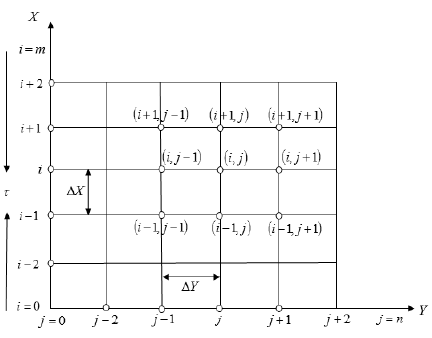Figure 1(a). The finite difference space grid

The constant mash size is wangled ΔX=0.83(0≤x≤125); ΔY=0.50(0≤y≤125) with the smaller time-step, Δ$\tau$=0.0005. Now,

$\frac{{{U}_{i,j}}-{{U}_{i-1,j}}}{\Delta X}+\frac{{{V}_{i,j}}-{{V}_{i,j-1}}}{\Delta Y}=0$ (14)

$\frac{{{{{U}'}}_{i,j}}-{{U}_{i,j}}}{\Delta \tau }+{{U}_{i,j}}\left( \frac{{{U}_{i,j}}-{{U}_{i-1,j}}}{\Delta X} \right)+{{V}_{i,j}}\left( \frac{{{U}_{i,j+1}}-{{U}_{i,j}}}{\Delta \Upsilon } \right)$

$=\left( 1+\frac{1}{\beta } \right)\left( \frac{{{U}_{i,j+1}}-2{{U}_{i,j}}+{{U}_{i,j-1}}}{{{\left( \Delta \Upsilon \right)}^{2}}} \right)+Gr{{\bar{T}}_{i,j}}\,+Gm{{\bar{C}}_{i,j}}-\left( 1+\frac{1}{\beta } \right)kp{{U}_{i,j}}-M{{U}_{i,j}}$ (15)

$\frac{{{{{\bar{T}}'}}_{i,j}}-{{{\bar{T}}}_{i,j}}}{\Delta \tau }+{{U}_{i,\,j}}\frac{{{{\bar{T}}}_{i,j}}-{{{\bar{T}}}_{i-1,j}}}{\Delta X}+{{V}_{i,\,j}}\frac{{{{\bar{T}}}_{i,j+1}}-{{{\bar{T}}}_{i,j}}}{\Delta Y}$

$=\frac{1}{\Pr }\left( 1+\frac{4}{3}Ra \right)\frac{{{{\bar{T}}}_{i,j+1}}-2{{{\bar{T}}}_{i,j}}+{{{\bar{T}}}_{i,j-1}}}{{{\left( \Delta Y \right)}^{2}}}+S\,{{\bar{T}}_{i,j}}\,$

$+\frac{1}{\Pr }\left( \frac{\lambda }{1+\lambda {{{\bar{T}}}_{i,j}}} \right){{\left( \frac{{{{\bar{T}}}_{i,j+1}}-{{{\bar{T}}}_{i,j}}}{\Delta \Upsilon } \right)}^{2}}+Nb$

$\left( \frac{{{{\bar{C}}}_{i,j+1}}-{{{\bar{C}}}_{i,j}}}{\Delta Y} \right)\left( \frac{{{{\bar{T}}}_{i,j+1}}-{{{\bar{T}}}_{i,j}}}{\Delta Y} \right)+Nt{{\left( \frac{{{{\bar{T}}}_{i,j+1}}-{{{\bar{T}}}_{i,j}}}{\Delta Y} \right)}^{2}}$ (16)

$\frac{{{{{\bar{C}}'}}_{i,j}}-{{C}_{i,j}}}{\Delta \tau }+{{U}_{i,\,j}}\frac{{{{\bar{C}}}_{i,j}}-{{{\bar{C}}}_{i-1,j}}}{\Delta X}+{{V}_{i,\,j}}\frac{{{{\bar{C}}}_{i,j+1}}-{{{\bar{C}}}_{i,j}}}{\Delta Y}$

$=\left( \frac{1}{Sc}+\frac{1}{Le} \right)\frac{{{{\bar{C}}}_{i,j+1}}-2{{{\bar{C}}}_{i,j}}+{{{\bar{C}}}_{i,j-1}}}{{{\left( \Delta Y \right)}^{2}}}\,\,-\gamma {{\bar{C}}_{i,\,j}}+\left( Sr+\frac{{{N}_{t}}}{NbLe} \right)\frac{{{{\bar{T}}}_{i,j+1}}-2{{{\bar{T}}}_{i,j}}+{{{\bar{T}}}_{i,j-1}}}{{{\left( \Delta Y \right)}^{2}}}$ (17)

The intermeddle boundary periphery in explicit form as:

\left. \begin{align} & \tau >0,\,\,\,U_{i,\,0}^{n}=\text{e}{{\,}^{(an\Delta \tau )}},\,\,V_{i,\,0}^{n}=0,\,\bar{T}_{i,\,0}^{n}=\text{e}{{\,}^{(n\Delta \tau )}},\,\, \\ & \,\,\,\,\,\,\,\,\,\,\,\,\,\,\,\,\,\,\,\,\,\,\,\,\,\,\,\,\,\,\,\,\,\,\,\,\,\bar{C}_{i,\,0}^{n}=\text{e}{{\,}^{(n\Delta \tau )}}\,\,\,\,\,\,\,\,\,\,\text{at}\,\,\text{Y}=\text{0} \\ & \,\,\,\,\,\,\,U_{i,\,L}^{n}=0,\,\,\,\bar{T}_{i,\,L}^{n}\to 0,\,\,\bar{C}_{i,\,L}^{n}\to 0\,\,\text{where}\,\,L\to \infty \\ \end{align} \right\} (18)

Here i and j designate to the mesh points with X and Y coordinate respectively and the superscripts n connote a value of time, t=nΔ$\tau$ where, n=0, 1, 2 ….

4. Stability and Convergence Analysis

The exploration persists deficient without the stability and convergence test (SCT) as long as the finite difference scheme has been exerted. For the explicit mesh sizes, the stability criteria of the scheme has been actuated as follows:

The equation (14) has been eradicated because Δ$\tau$ does not transpire in it. The quotidian terms of the Fourier expansion for $U,\,\,\bar{T}\,\text{and}\,\,\bar{C}$ at a logically time $\tau$=0 are all eiaX eibY aloof form a constant, $i=\sqrt{-1}$. At a time $\tau$, these terms become:

\left. \begin{align} & U:\,\,\,\,\,\,\,\,A(\tau ){{e}^{i\alpha X}}{{e}^{i\beta Y}} \\ & \bar{T}:\,\,\,\,\,\,\,\,C(\tau ){{e}^{i\alpha X}}{{e}^{i\beta Y\,\,\,\,\,}} \\ & \bar{C}:\,\,\,\,\,\,\,\,D(\tau ){{e}^{i\alpha X}}{{e}^{i\beta Y}} \\ \end{align} \right\} (19)

After the time step,

\left. \begin{align} & U:\,\,\,\,\,\,\,\,{A}'(\tau ){{e}^{i\alpha X}}{{e}^{i\beta Y}} \\ & \bar{T}:\,\,\,\,\,\,\,\,{C}'(\tau ){{e}^{i\alpha X}}{{e}^{i\beta Y\,\,\,\,\,}} \\ & \bar{C}:\,\,\,\,\,\,\,\,{D}'(\tau ){{e}^{i\alpha X}}{{e}^{i\beta Y}} \\ \end{align} \right\} (20)

By cast away the above values the following palliation equations are erected as:

$\frac{({A}'-A){{e}^{i\alpha X}}{{e}^{i\beta Y}}}{\Delta \tau }+\,U\frac{A{{e}^{i\alpha X}}{{e}^{i\beta Y}}(1-{{e}^{-i\alpha \Delta X}})}{\Delta X}+V\,\frac{A{{e}^{i\alpha X}}{{e}^{i\beta Y}}({{e}^{i\beta \Delta Y}}-1)}{\Delta Y}=\,\left( 1+\frac{1}{\beta } \right)$

$\frac{2A{{e}^{i\alpha X}}{{e}^{i\beta Y}}(\cos \beta \Delta Y-1)}{{{(\Delta Y)}^{2}}}\,+GrC{{e}^{i\alpha X}}{{e}^{i\beta Y}}+GmDe^{i\alpha X}{{e}^{i\beta Y}}-\left( 1+\frac{1}{\beta } \right)KpA{{e}^{i\alpha X}}{{e}^{i\beta Y}}-M\,A{{e}^{i\alpha X}}{{e}^{i\beta Y}}$ (21)

$\frac{({C}'-C){{e}^{i\alpha X}}{{e}^{i\beta Y}}}{\Delta \tau }+\,U\frac{C{{e}^{i\alpha X}}{{e}^{i\beta Y}}(1-{{e}^{-i\alpha \Delta X}})}{\Delta X}+V\,\frac{C{{e}^{i\alpha X}}{{e}^{i\beta Y}}({{e}^{i\beta \Delta Y}}-1)}{\Delta Y}=\frac{1}{\Pr }\left( 1+\frac{4}{3}Ra \right)$

$+Nb\frac{D{{e}^{i\alpha X}}{{e}^{i\beta Y}}(1-{{e}^{-i\alpha \Delta X}})}{\Delta X}\frac{C{{e}^{i\alpha X}}{{e}^{i\beta Y}}({{e}^{i\beta \Delta Y}}-1)}{\Delta Y}$ (22)

$\frac{({D}'-D){{e}^{i\alpha X}}{{e}^{i\beta Y}}}{\Delta \tau }+\,U\frac{D{{e}^{i\alpha X}}{{e}^{i\beta Y}}(1-{{e}^{-i\alpha \Delta X}})}{\Delta X}+V\,\frac{D{{e}^{i\alpha X}}{{e}^{i\beta Y}}({{e}^{i\beta \Delta Y}}-1)}{\Delta Y}=\left( \,\frac{1}{Sc}+\frac{1}{Le} \right)$

$\left[ \frac{2D{{e}^{i\alpha X}}{{e}^{i\beta Y}}(\cos \beta \Delta Y-1)}{{{(\Delta Y)}^{2}}} \right]+\left( Sr+\frac{Nt}{NbLe} \right)\left[ \frac{2C{{e}^{i\alpha X}}{{e}^{i\beta Y}}(\cos \beta \Delta Y-1)}{{{(\Delta Y)}^{2}}} \right]-\gamma \,D{{e}^{i\alpha X}}{{e}^{i\beta Y}}$ (23)

The overhead equations are revealed as

${A}'={{A}_{1}}A+{{A}_{2}}C+{{A}_{3}}D$ (24)

${C}'={{A}_{4}}C$ (25)

${D}'={{A}_{5}}D+{{A}_{6}}C$ (26)

where,

${{A}_{1}}=1+\left( 1+\frac{1}{\beta } \right)\frac{2\Delta \tau }{{{(\Delta Y)}^{2}}}(\cos \beta \Delta Y-1)-U\frac{\Delta \tau }{\Delta X}(1-{{e}^{-i\alpha \Delta X}})-V\,\frac{\Delta \tau }{\Delta Y}({{e}^{i\beta \Delta Y}}-1)-\left( 1+\frac{1}{\beta } \right)Kp\,\Delta \tau -M\Delta \tau$

${{A}_{2}}=\,Gr\Delta \tau$; ${{A}_{3}}=\,Gm\Delta \tau$

${{A}_{4}}=1+\frac{1}{\Pr }\left( 1+\frac{4}{3}Ra \right)\frac{2\Delta \tau }{{{(\Delta Y)}^{2}}}(\cos \beta \Delta Y-1)-U\frac{\Delta \tau }{\Delta X}(1-{{e}^{-i\alpha \Delta X}})-V\,\frac{\Delta \tau }{\Delta Y}({{e}^{i\beta \Delta Y}}-1)+S\Delta \tau$

$+\frac{1}{\Pr }\left( \frac{\lambda }{1+\lambda \overline{C}} \right)\frac{2\Delta \tau }{{{(\Delta Y)}^{2}}}(\cos \beta \Delta Y-1)-U\frac{\Delta \tau }{\Delta X}(1-{{e}^{-i\alpha \Delta X}})-V\,\frac{\Delta \tau }{\Delta Y}({{e}^{i\beta \Delta Y}}-1)+S\Delta \tau$

${{A}_{5}}=1+\,\left( \,\frac{1}{Sc}+\frac{1}{Le} \right)\frac{2\Delta \tau }{{{(\Delta Y)}^{2}}}(\cos \beta \Delta Y-1)-U\frac{\Delta \tau }{\Delta X}(1-{{e}^{-i\alpha \Delta X}})-V\,\frac{\Delta \tau }{\Delta Y}({{e}^{i\beta \Delta Y}}-1)-\gamma \Delta \tau$

${{A}_{6}}=\left( Sr+\frac{Nt}{NbLe} \right)\frac{2\Delta \tau }{{{(\Delta Y)}^{2}}}(\cos \beta \Delta Y-1)$

Therefore the matrix form is:

$\,\left[ \begin{matrix} {{A}'} \\ {{C}'} \\ {{D}'} \\ \end{matrix} \right]=\left[ \begin{matrix} {{A}_{1}} & {{A}_{2}} & {{A}_{3}} \\ 0 & {{A}_{4}} & 0 \\ 0 & {{A}_{6}} & {{A}_{5}} \\ \end{matrix} \right]\left[ \begin{matrix} A \\ B \\ C \\ \end{matrix} \right]$ (27)

Therefore, ${\eta }'={T}'\eta$, where:

$\eta '=\left[ \begin{matrix} A' \\ C' \\ D' \\ \end{matrix} \right]$

${T}'=\left[ \begin{matrix} {{A}_{1}} & {{A}_{2}} & {{A}_{3}} \\ 0 & {{A}_{4}} & 0 \\ 0 & {{A}_{6}} & {{A}_{5}} \\ \end{matrix} \right];$ $\eta =\left[ \begin{matrix} A \\ B \\ C \\\end{matrix} \right]$.

This is a fourth order square matrix. So, it is very hard to find out the eigenvalues and Δ$\tau$ is very compact in EFDM, i.e. Δ$\tau\to$ 0. Under this appraisal, A2$\to$0, A3$\to$0 and A6$\to$0.

Therefore,

${T}'=\left[ \begin{matrix} {{A}_{1}} & 0 & 0 \\ 0 & {{A}_{4}} & 0 \\ 0 & 0 & {{A}_{5}} \\\end{matrix} \right];$ (28)

Therefore the eigenvalues are retrieved as l1=A1, l2=A4 and l3=A5 such that $\left| {{A}_{1}} \right|\le 1\,\,\,\left| {{A}_{4}} \right|\le 1\text{and}\,\left| {{A}_{5}} \right|\le 1\,$ and convinced admissible values are A1=-1, A4=-1 and A5=-1.

So, the stability conditions are:

$(1+1/β) 2Δτ/((ΔY)^2 )+MΔτ/2+U Δτ/ΔX+|-V| Δτ/ΔY+\left( 1+\frac{1}{\beta } \right)\frac{Kp\Delta \tau }{2}\le 1$ (29)

$\frac{1}{\Pr }\left( 1+\frac{4}{3}Ra \right)\frac{2\Delta \tau }{{{(\Delta Y)}^{2}}}+\left( \frac{1}{\Pr }+Nt \right){{\left( \frac{\Delta \tau }{\Delta Y} \right)}^{2}}$

$+U\frac{\Delta \tau }{\Delta X}+\left| -V \right|\,\frac{\Delta \tau }{\Delta Y}-\frac{S\,\Delta \tau }{2}\le 1$ (30)

$\left( \frac{1}{Sc}+\frac{1}{Le} \right)\frac{2\Delta \tau }{{{(\Delta Y)}^{2}}}+\frac{\gamma \Delta \tau }{2}+U\frac{\Delta \tau }{\Delta X}+\left| -V \right|\,\frac{\Delta \tau }{\Delta Y}\le 1$ (31)

Exploiting basic agreement, U=V=T=C=0 at t=0 the convergence criteria of the existent problem are retrieved as Sc$\ge$0.18 and Le$\ge$0.20.

5. Results and Discussion

The numerical computations have been manipulated for the disparate values of miscellaneous parameters by an explicit finite difference method (EFDM) for the aspiration of scrutinizing the upshots of the contemporary work. The physical stipulation of the contemporaneous model such that velocity, temperature, concentration, skin friction coefficient, Nusselt number, Sherwood number, streamlines and isotherms lines has been procured for distinct parameters which are indicated in Figures 2 to 23. In order to prevail the numerical results, the succeeding values of default parameter are deputed as: M=1.0, Pr=0.71, Sc=0.22, Ra=0.50, $\beta$=0.40, S=0.1, Nb=0.5, Nt =0.8, Le=2.5, Sr=1, Gm=5, $\lambda$=2, Gr=10, Kp=1.0, and $\gamma$=0.50 with time $\tau$=10. These values are critiqued as homogeneous throughout the present study in respective figures.

## 2.png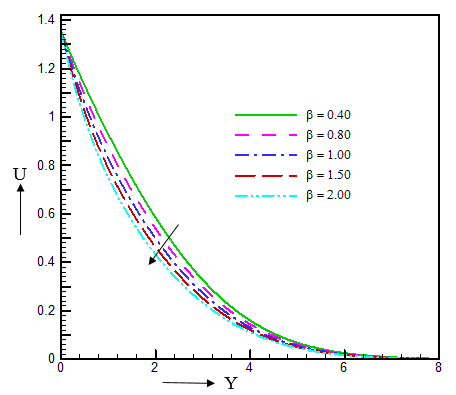Figure 2. The illustration of $\beta$ on U

## 3.png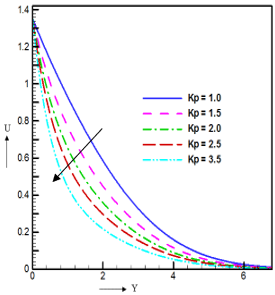Figure 3. The illustration of Kp on U

The impression of separate values of b and Kp on velocity are elucidated in Figure 2 and Figure 3, respectively. It is proclaimed that velocity are economized 13.84 %, 9.01 %, 20.8 %, and 19.92 % by $\beta$=0.40 to $\beta$=0.80, $\beta$=0.80 to $\beta$=1.00, $\beta$=1.00 to $\beta$=1.50 and $\beta$=1.50 to $\beta$=2.00 at Y=0.4556. Physically, by elaborating the values of b which remises the yield stress suppresses in the fluid velocity. Also, velocity is extenuation due to the prolongation of Kp which ensues in Figure 3. Naturally, permeability of porous medium which propagated a resistive force in the fluid. The numerical evolution of isolated parameters of U are flaunted in the Table 1 and Figures 2-3.

Table 1. Delineates the curve to curve fluid velocity (Figures. 2, 3) at Y= 0.4556 and $\eta$=10

 Values of Parameter Numerical Value Increase/Decrease in (%) $\beta$=0.40 0.13561 Initial position $\beta$=0.80 0.11684 13.84% decrease $\beta$=1.00 0.10632 9.01% decrease $\beta$=1.50 0.08413 20.87% decrease $\beta$=2.00 0.06737 19.92% decrease Kp=1.00 0.13561 Initial position Kp=1.50 0.10002 26.24% decrease Kp=2.00 0.07755 22.47% decrease Kp=2.50 0.06311 18.62% decrease Kp=3.50 0.04589 27.29% decrease

## 4.png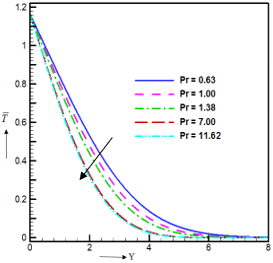Figure 4. The illustration of Pr $\bar{T}\,$

The impression of Pr, Nb, Ra and S on temperature schemes are found in the Figures 4-7. It is worth mentioned that temperature allocations are collapsed due to the enhancement of Pr. Instinctively, high Prandtl number have higher viscosity, which constricted the thermal boundary layer depth. Also, the temperature delineations are retrenched considerable with the accrual of Nb because it innovate an unbridled motions in the fluid. Here, temperature depictions are protracted as 35.46 %, 16.02 %, 9.84 %, and 7.06 % for Ra=0.50 to Ra=1.00, Ra=1.00 to Ra=1.50, Ra=1.50 to Ra=2.00 and Ra=2.00 to Ra=2.50 because the thermal boundary layer thickness are prosecuted due to completion of Ra. Further, temperature disbursements are surged due to augmentation of S. The preeminent vindication behind this upshot is that the heat absorption invokes a drop in the kinetic energy as well as thermal energy of the fluid. The numerical disposition of relative parameters are evinced in the Table 2 and Figures 4-7.

## 6.png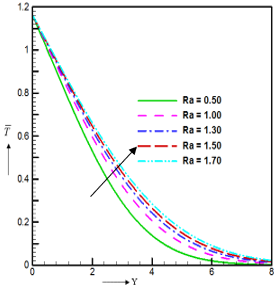Figure 5. The illustration of Nt on $\bar{T}\,$

## 5.png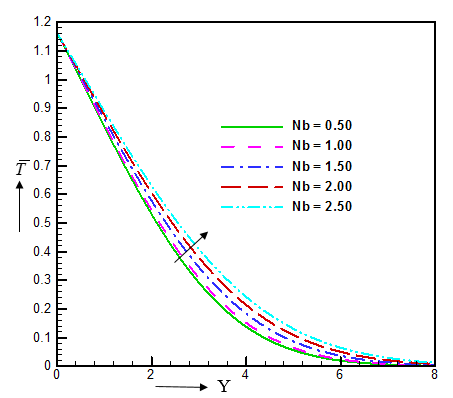Figure 6. The illustration of Ra on $\bar{T}\,$

## 7.png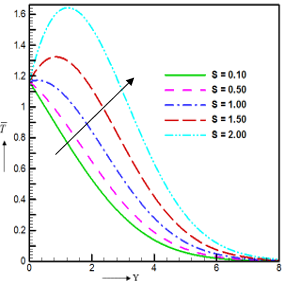Figure 7. The illustration of S on $\bar{T}\,$

The denouements of $\gamma$, Sc, Le and Nb on concentration propagations are eliciting in Figures 8-11. By the offshoot of chemical reaction, concentration is alleviated because the positive values of chemical reaction parameter ($\gamma$>0) presages the sequels of destructive chemical reaction on the concentration field. It is fact that, Sc and Le constricts the concentration. It is true by physically that Schmidt number aggrandizes the density of the fluid as well as concentration buoyancy force in the fluid. Also, from Figure 11, we perceived that concentration disposals abridge to the amplifications of Nb which leads the concentration allocation. Physically, it is intimated that Nt elongates the nanoparticle diffusion. The numerical values of these are dispensed in the Table 3 and Figures 8-11.

The Figures 12 to 17 epitomize the repercussions of $\beta$, Ra, Gr and Pr on skin friction coefficient (Cf), Nusselt number (Nu) and Sherwood number(Sh) for Casson nanofluid. It is devised that, Cf protracts with the accession of $\beta$ and $\gamma$ which is disported in Figure 12 and Figure 13 respectively. Physically, skin friction is aggrandized the rate of velocity of the fluid flow. On the other hand the Nusselt number is a decay function of Ra and Gr but proliferation function of Pr. Generally, convective heat transfer distends with the quadrupling of Nusselt number. Also it is articulated from Figure 17 that, the Sherwood number prolongs with the retrenchment of Grashof number (Gr).

Table 2. Incarnates the curve to curve fluid temperature (Figures 4-7) at Y= 4.18060 and $\tau$=10.0

 Values of Parameter Numerical Value Increase/Decrease in (%) Pr=0.63 0.11834 Initial position Pr=1.00 0.10988 7.14% decrease Pr=1.38 0.09093 17.24% decrease Pr=7.00 0.05362 41.03% decrease Pr=11.62 0.05030 6.19% decrease Ra=0.50 0.11834 Initial position Ra=1.00 0.18336 35.46% increase Ra=1.50 0.21834 16.02% increase Ra=2.00 0.24216 9.84% increase Ra=2.50 0.26056 7.06% increase Nb=0.50 0.11834 Initial position Nb=1.00 0.13127 9.85% increase Nb=1.50 0.16192 18.93% increase Nb=2.00 0.19097 15.31% increase Nb=2.50 0.21830 12.52% increase S=0.10 0.11834 Initial position S=0.50 0.16319 27.48% increase S=1.00 0.24429 33.19% increase S=1.50 0.36841 33.79% increase S=2.00 0.56075 34.33% increase

## 8.png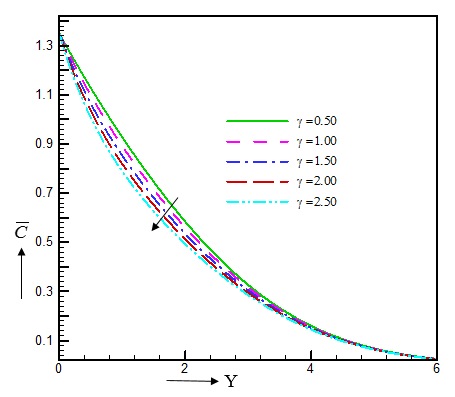Figure 8. The illustration of $\lambda$ on $\bar{C}$

## 9.png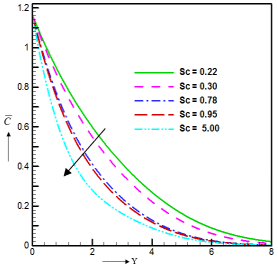Figure 9. The illustration of Sc on$\bar{C}$

## 10.png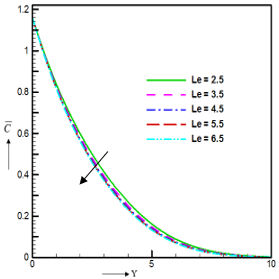Figure 10. The illustration of Le on $\bar{C}$

## 11.png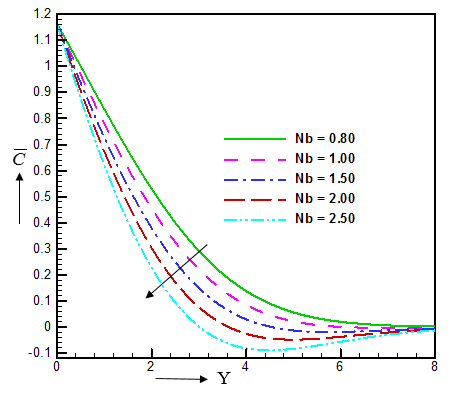Figure 11. The illustration of Nt on $\bar{C}$

Table 3. Emblematizes the curve to curve fluid concentration (Figures 8-11) at Y= 4.18060 and $\tau$=10.0

 Values of parameter Numerical value Increase/decrease in (%) $\gamma$=0.50 0.24736 Initial position $\gamma$==1.00 0.19056 22.96% decrease $\gamma$==1.50 0.14917 21.72% decrease $\gamma$==2.00 0.11864 20.47% decrease $\gamma$==2.50 0.09583 19.22% decrease Le=2.50 0.24736 Initial position Le=3.50 0.23075 6.71% decrease Le=4.50 0.22136 4.06% decrease Le=5.50 0.21532 2.73% decrease Le=6.50 0.21111 1.96% decrease Sc=0.22 0.24736 Initial position Sc=0.30 0.20128 33.97% decrease Sc=0.78 0.11386 18.62% decrease Sc=0.95 0.10479 43.43% decrease Sc=5.00 0.07891 24.79% decrease Nb=0.80 0.24736 Initial position Nb=1.00 0.20355 17.71% decrease Nb=1.80 0.19804 2.71% decrease Nb=2.00 0.18124 8.48% decrease Nb=2.50 0.15329 15.82% decrease

## 12.png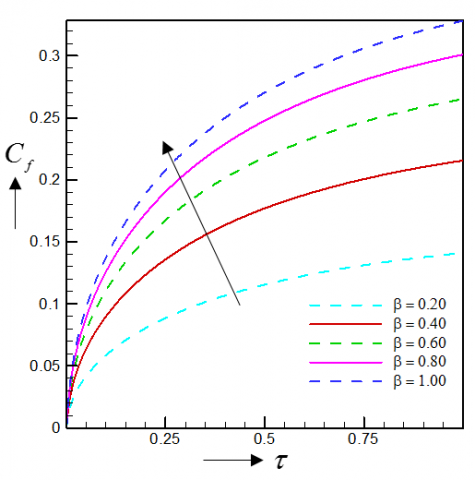Figure 12. Skin friction for different values of $\beta$

## 13.png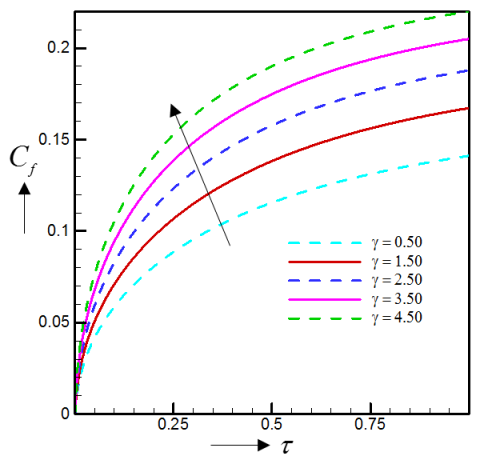Figure 13. Skin friction for different values of $\gamma$

## 14.png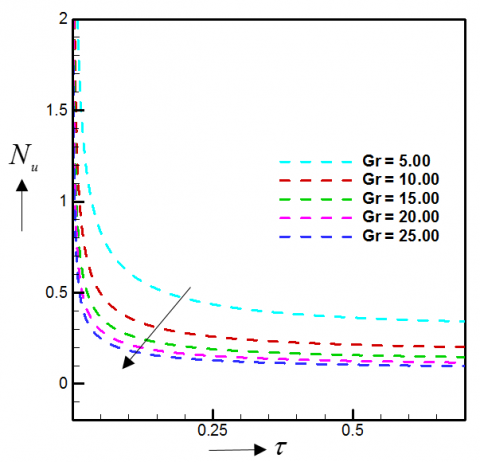Figure 14. Nusselt number for different values of Gr

## 15.png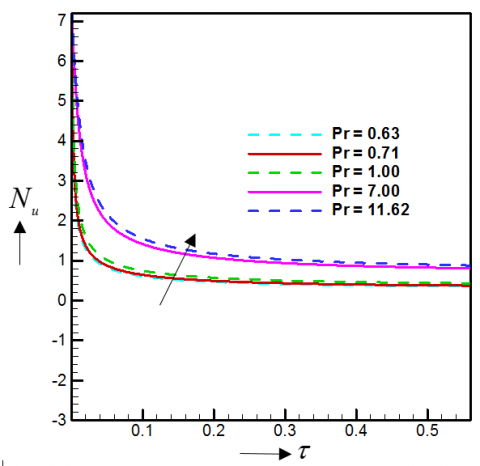Figure 15. Nusselt number for different values of Pr

## 16.png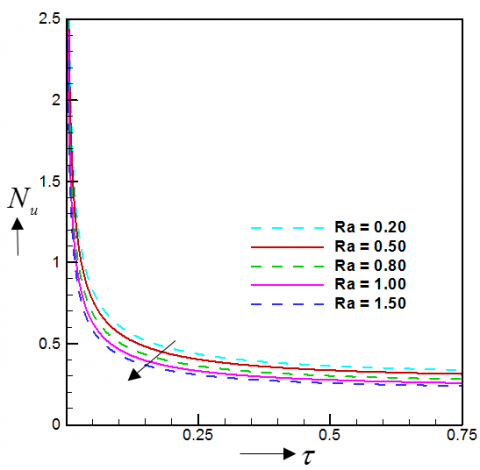Figure 16. Nusselt number for different values of Ra

## 17.png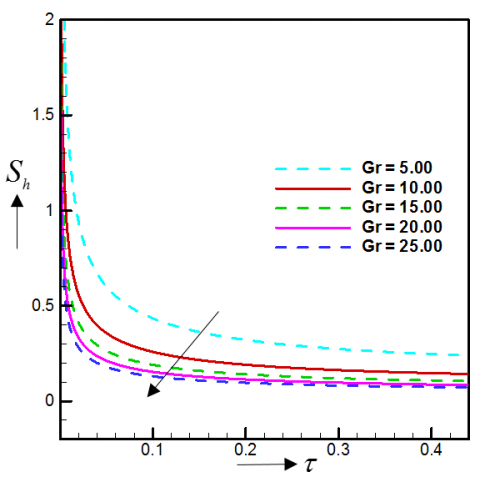Figure 17. Sherwood number for different values of Gr

## 18.png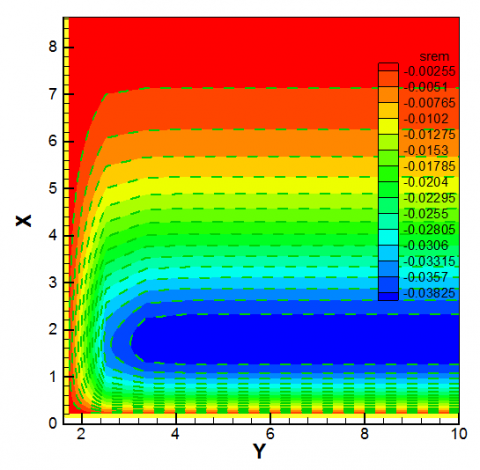Figure 18. Illustration of Streamlines for Ra=0.50 and Ra=1.50

## 19.pngFigure 19. Illustration of Isotherms for Ra=0.50 and Ra=1.50
The dimensionless equations are unravelled numerically by EFDM such that, X and Y axis are dimensionless which depicts the mash point. Also, the stream and isotherms curves are disported in the above prominent Figures. Here, legend values of stream and isotherms insinuate the contours levels. Furthermore, streamlines dispensations can be utilized to meliorate the visualization of fluid fields. The boundary layer system of change can be proclaimed by an isotherm, where the temperature endures constant (ΔT=0). An isotherm at 0 ℃ is denominated the freezing level. The denouement of radiation parameter (Ra) on streamlines and isotherms are construed in Figure 18 and Figure 19. The momentum boundary layer and thermal boundary layer inflate due to the escalation of Ra. Also, Figure 19 evinces the thermal direction of fluid with the comportment of two distinct thermal radiation parameters. The isotherms plot of the momentum boundary layer thickness with the poise of Nb where the green dashed flood lines is for Nb=0.50 and Nb=2.50 is trumped in Figure 20. Figure 21 struts the contour flood view for thermal Brownian motion parameter difference. The legend values of Figure 21 are given in the contours levels. Here we perceived that the thermal and momentum boundary layer thickness is augmented due to addendum of Nb.

## 20.png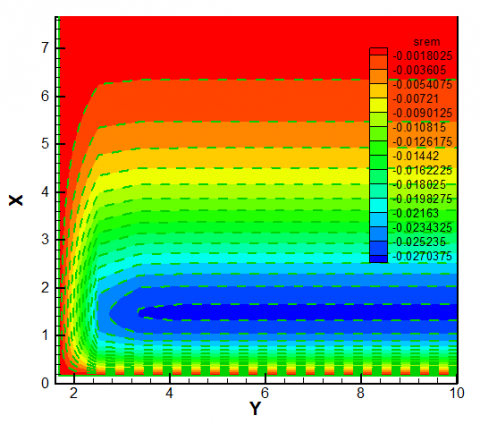Figure 20. Illustration of Streamlines for Nb=0.50 and Nb=2.50

## 21.pngFigure 21. Illustration of Isotherms for Nb=0.50 and Nb=2.50

## 22.png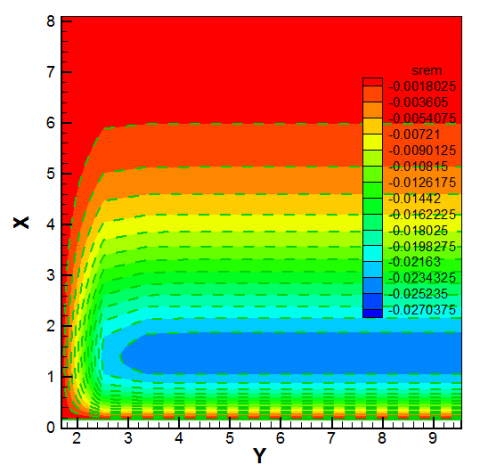Figure 22. Illustration of Streamlines for Nt=0.80 and Nt=1.30

## 23.png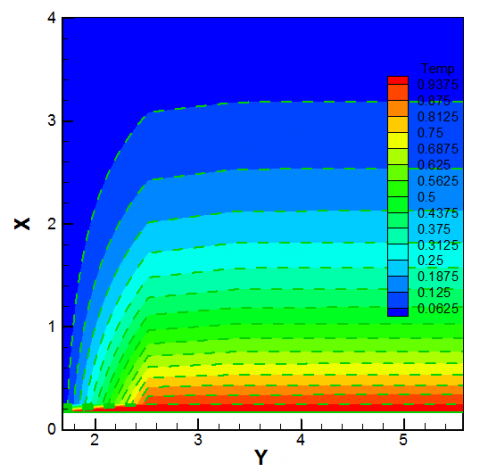Figure 23. Illustration of Isotherms for Nt=0.80 and Nt=1.30
The momentum and thermal direction of fluid (thermal boundary layer thickness) with divergent Nt are flaunted in the Figure 22 and 23. Figure 22 divulges the contour flood view of momentum boundary layer and Figure 23 imparts the thermal boundary layer thickness with legend values which are the contours levels. In this apprehension we have discerned that the momentum boundary layer thickness is escalating but thermal boundary layer is abbreviating due to the propagation of Nt.
6. Conclusion

In this disquisition, the unsteady MHD heat and mass transfer of Casson nanofluid flow in the appearance of variable thermal conductivity, heat absorption and thermal radiation has been propounded. The predicament is evaluated under the influence of strong cross field and consideration has been endorsed to those pragmatic fluids which are altered as liquid metals. The governing model equations are elucidated by utilizing explicit finite difference method (EFDM) and the numerical results are enumerated for the divergent dimensionless parameters. In this observation the Casson parameter and permeability of porous medium abates the velocity profiles. The Nusselt number is plunged by radiation parameter and Grashof number but accumulated by Prandtl number. Also, the skin friction coefficient proliferates with the inflation of Casson parameter and chemical reaction parameter. Further, Brownian motion parameter (Nb), chemical reaction (g), Schmidt number (Sc) and Lewis number (Le) wanes the concentration profiles. But, Brownian motion parameter (Nb) which elevates the temperature profiles. Furthermore, temperature depictions are aggravating due to the amplification of heat source parameter and radiation parameter but dwindling due to the amelioration of Prandtl number.

Table 4. The previous results by Ghadikolaei et al. 

 Previous Results by Ghadikolaei et al.  Increased Parameters U $\bar{T}\,$ $\bar{C}\,$ Skin fri. Nusl. Num. Sher. Num. Gr Inc Nb Inc Dec b Dec Inc Inc S Inc Inc R Inc Inc g Dec Inc Pr Dec Le Dec

Table 5. Comparison of the accuracy of the present results with the previous results by Ghadikolaei et al. 

 Previous Results by Ghadikolaei et al.  Increased Parameters U $\bar{T}\,$ $\bar{C}\,$ Skin fri. Nusl. Num. Sher. Num. Gr Nb Inc Dec b Dec S Inc Inc R Inc Dec g Dec Pr Dec Le Dec

Comparison of the accuracy of the present results with the previous results by Ghadikolaei et al.  are impersonated in the above Table 4. Here, it is permitted that, all values are same without just only one result is isolated with the works of Ghadikolaei et al. . In this present disquisition, Nusselt number is dwindled by radiation parameter but opposite with the work of Ghadikolaei et al. .

Nomenclature
 x,y Cartesian coordinates (m) u,v Velocity components (m/s) $\gamma$ Chemical reaction parameter (-) U Dimensionless primary velocity (m/s) Sc Schmidt number (-) Nu Nusselt number (-) S Heat source parameter (-) Cf Skin friction (-) $\rho$ Fluid density (kg/m3) v Kinematic viscosity (m2s-1) Tm Mean fluid temperature (K) Cs Concentration susceptibility (J/kg·K) Sh Sherwood number (-) $\bar{T}\,$ Dimensionless fluid temperature (K) Nt Thermophoresis parameter (-) $\lambda$ Thermal conductivity variation parameter (-) g Acceleration due to gravity (ms-2) M Magnetic parameter (Wbm-2) bT Thermal expansion coefficient (-) Pr Prandlt number (-) Gr Grashof number (-) Gm Modified Grashof number (-) Kp Permeability of porous medium (-) b Casson parameter (-) Sr Soret number (-) T Temperature of fluid (K) k Thermal conductivity (W/m·K) Cp Specific heat at constant pressure (J/m3·K) bc Concentration expansion coefficient (-) $\bar{C}\,$ Dimensionless fluid concentration (-) Le Lewis number (-) Nb Brownian motion parameter (-) Dm Molecular diffusivity of the species concentration (-) σ Stefan-Boltzmann constant (W/m2 K4)
References

 Oyelakin IS, Mondal S, Sibanda P. (2016). Unsteady Casson nanofluid flow over a stretching sheet with thermal radiation, convective and slip boundary conditions. Alexandria Engineering Journal 55: 1025-1035. https://doi.org/10.1016/j.aej.2016.03.003

 Kataria HR, Patel HR. (2016). Soret and heat generation effects on MHD Casson fluid flow past an oscillating vertical plate embedded through porous medium. Alexandria Engineering Journal 55: 2125-2137. https://doi.org/10.1016/j.aej.2016.06.024

 Ahmed N, Khan U, Khan SI, Bano S, Mohyud-Din ST. (2017). Effects on magnetic field in squeezing flow of a Casson fluid between parallel plates. Journal of King Saud University Science 29: 119-125. https://doi.org/10.1016/j.jksus.2015.03.006

 Mahanta G, Shaw S. (2016). 3D Casson fluid flow past a porous linearly stretching sheet with convective boundary condition. Alexandria Engineering Journal 54: 653-659. https://doi.org/10.1016/j.aej.2015.04.014

 Afikuzzaman M, Ferdows M, Alam MM. (2015). Unsteady MHD Casson fluid flow through a parallel plate with Hall current. Procedia Engineering 105: 287-293. https://doi.org/10.1016/j.proeng.2015.05.111

 Biswas R, Mondal M, Sarkar DR, Ahmmed SF. (2017). Effects of radiation and chemical reaction on MHD unsteady heat and mass transfer of Casson fluid flow past a vertical plate. Journal of Advances in Mathematics and Computer Science 23(2): 1-16. http://www.sciencedomain.org/review-history/19926

 Afikuzzaman M, Alam MM. (2016). MHD casson fluid flow through a parallel plate. Thammasat International Journal of Science and Technology 21(1): 1-7. https://tci-thaijo.org/index.php/SciTechAsia/52122

 Wahiduzzaman M, Islam MT, Sultana P, Afikuzzaman M. (2014). MHD couette flow of a Casson fluid between parallel porous plates. Progress in Nonlinear Dynamics and Chaos 2(2): 51-60.

 Biswas R, Ahmmed SF. (2018). Effects of Hall current and chemical reaction on MHD unsteady heat and mass transfer of Casson nanofluid flow through a vertical plate. Journal of Heat Transfer 140: 092402-1.

 Khalid A, Khan I, Khan A, Shafie S. (2015). Unsteady MHD free convection flow of casson fluid past over an oscillating vertical plate embedded in a porous medium. Engineering Science and Technology 18: 309-317. https://doi.org/10.1016/j.jestch.2014.12.006

 Ali ME, Sandeep N, (2017). Cattaneo-christov model for radiative heat transfer of magneto-hydrodynamic casson-ferrofluid: A numerical study. Results in Physics 7: 21-30. https://doi.org/10.1016/j.rinp.2016.11.055

 Ghadikolaei SS, Hosseinzadeh KH, Ganji DD, Jafari B. (2018). Nonlinear thermal radiation effect on magneto Casson nanofluid flow with Joule heating effect over an inclined porous stretching sheet. Case Studies in Thermal Engineering 12: 176-187. https://doi.org/10.1016/j.csite.2018.04.009

 Chai YH, Yusup S, Soonc CV, Arpinc MT. (2016). Rheological behaviour of graphene Nano-sheets in hydrogenated oil-based drilling fluid. Procedia Engineering Journal 148: 49-56. https://doi.org/10.1016/j.proeng.2016.06.490

 Ganga B, Ansari SMY, Ganeshc NV, Hakeem AKA. (2016). MHD flow of Boungiorno model nanofluid over a vertical plate with internal heat generation/absorption. Propulsin and Power Research 5(3): 211-222. https://doi.org/10.1016/j.jppr.2016.07.003

 Khan NA, Sultan F, Rubbab Q. (2015). Optimal solution of nonlinear heat and mass transfer in a two-layer flow with nano-Eyring–Powell fluid. Results in Physics 5: 199–205. https://doi.org/10.1016/j.rinp.2015.08.006

 Biswas P, Arifuzzaman SM, Karim I, Khan MS. (2017). Impacts of magnetic field and radiation absorption on mixed convective Jeffrey nano fluid flow over a vertical stretching sheet with stability and convergence analysis. Journal of Nanofluid 6(6): 1082-1095. https://doi.org/10.1166/jon.2017.1407

 Bég OA, Khan MS, Karim I, Alam MM., Ferdows M. (2014). Explicit numerical study of unsteady hydromagnetic mixed convective nanofluid flow from an exponentially stretching sheet in porous media. Applied Nanoscience 4: 943-957.

 Dogonchi AS, Divsalar K, Ganji DD. (2016). Flow and heat transfer of MHD nanofluid between parallel plates in the presence of thermal radiation. Computer Methods in Applied Mechanics and Engineering 310: 58-76. https://doi.org/10.1016/j.cma.2016.07.003

 Dogonchi AS, Alizadeh M, Ganji DD. (2017). Investigation of MHD go-water nanofluid flow and heat transfer in a porous channel in the presence of thermal radiation effect. Advanced Powder Technology 28(7): 1815-1825. https://doi.org/10.1016/j.apt.2017.04.022

 Ahmmed SF, Biswas R, Afikuzzaman M. (2018). Unsteady magnetohydrodynamic free convection flow of nanofluid through an exponentially accelerated inclined plate embedded in a porous medium with variable thermal conductivity in the presence of radiation. Journal of Nanofluids 7: 891-901. https://doi.org/10.1166/jon.2018.1520

 Leekea GA, Tiejun L, Bridsona RH, Jonathan PKS. (2016). Application of nano-particle coatings to carrier particles using an integrated fluidized bed supercritical fluid precipitation process. Journal of Supercritical Fluids 91: 7-14. https://doi.org/10.1016/j.supflu.2014.03.012

 Uddin MJ, Kalbani KSA, Rahman MM, Alam MS, Eltayed IA. (2016). Fundamentals of nanofluids: evolution, applications and new theory. Official Journal of Biomathematical Society of India 2(1): 1-32.

 Bilal S, Rehman KU, Malik MY, Hussain A, Awais M. (2017). Effect logs of double diffusion on MHD Prandtl nanofluid adjacent to 4 stretching surface by way of numerical approach. Results in Physics 7: 470–479. https://doi.org/10.1016/j.rinp.2016.11.008

 Arani AG, Zarei MS. (2015). Nonlocal vibration of y-shaped CNT conveying nano-magnetic viscous fluid under magnetic field. Ain Shams Engineering Journal 6: 565-575. https://doi.org/10.1016/j.asej.2014.11.012

 Murthy MVR, Raju RS, Rao JA. (2015). Heat and mass transfer effects on MHD natural convective flow past an infinite vertical porous plate with thermal radiation and Hall current. Procedia Engineering 127: 1330-1337. https://doi.org/10.1016/j.proeng.2015.11.491

 Das S, Jana RN, Makinde OD. (2015). Magnetohydrodynamic mixed convective slip flow over an inclined porous plate with viscous dissipation and joule heating. Alexandria Engineering Journal 54(2): 251-261. https://doi.org/10.1016/j.aej.2015.03.003

 Shit GC, Haldar R, Ghosh SK. (2016). Convective heat transfer and MHD viscoelastic nanofluid flow induced by a stretching sheet. International Journal of Applied and Computational Mathematics 2(59): 3–608.

 Ibrahim W. (2016). The effect of induced magnetic field and convective boundary condition on MHD stagnation point flow and heat transfer of upper-convected Maxwell fluid in the presence of nanoparticle past a stretching sheet. Propulsin and Power Research 5(2): 164-175. https://doi.org/10.1016/j.jppr.2016.05.003

 Rani HP, Kim CN. (2010). A numerical study on unsteady natural convection of air with variable viscosity over an isothermal vertical cylinder. Korean Journal of Chemical Engineering 27: 759-765. https://doi.org/10.1007/s11814-010-0211-x

 Animasaun IJ, Oyem AO. (2014). Effect of variable viscosity, Dufour, soret and thermal conductivity on free convection heat and mass transfer of non-Darcian flow past porous flat surface. American Journal of Computational Mathematics 33: 271-292. https://doi.org/10.1016/j.jnnms.2014.10.008

 Lavanya B, Ratnam AL. (2014). Dufour and soret effects on steady MHD free convective flow past a vertical porous plate embedded in a porous medium with chemical reaction, radiation, heat generation and viscous dissipation. Advances in Applied Science and Research 5(1): 127-142.

 Khan N, Mahmood T, Sajid M, Hashmi MS. (2016). Heat and mass transfer on MHD mixed convection axisymmetric chemically reactive flow of Maxwell fluid driven by exothermal and isothermal stretching disks. International Journal of Heat and Mass Transfer 92: 1090-1105. https://doi.org/10.1016/j.ijheatmasstransfer.2015.09.001

 Tripathy RS, Dash GC, Mishra SR, Baag S. (2015). Chemical reaction effect on MHD free convective surface over a moving vertical plate through porous medium. Alexandria Engineering Journal 54: 673-679. https://doi.org/10.1016/j.aej.2015.04.012

 Das S, Guchhait SK, Jana RN, Makinde OD. (2016). Hall effects on an unsteady magneto- convection and radiative heat transfer past a porous plate. Alexandria Engineering Journal 55: 1321-1331. https://doi.org/10.1016/j.aej.2016.04.027

 Dogonchi AS, Ganji DD. (2016). Thermal radiation effect on the nano-fluid buoyancy flow and heat transfer over a stretching sheet considering Brownian motion. Journal of Molecular Liquids 223: 521-527. https://doi.org/10.1016/j.molliq.2016.08.090

 Hsiao KL. (2017). Combined electrical MHD heat transfer thermal extrusion system using Maxwell fluid with radiative and viscous dissipation effects. Applied Thermal Engineering 112: 1281-1288. https://doi.org/10.1016/j.applthermaleng.2016.08.208

 Biswas R, Afikuzzaman M, Mondal M, Ahmmed SF. (2018). MHD free convection and heat transfer flow through a vertical porous plate in the presence of chemical reaction. Frontiers in Heat and Mass Transfer 11: 13. https://doi.org/10.5098/hmt.11.13

 Ahmmed SF, Biswas R. (2019). Effects of radiation and chemical reaction on MHD unsteady heat and mass transfer of nanofluid flow through a vertical plate. Modelling Measurement and Control B 87(4): 213-220.

 Biswas R, Mondal M, Islam A. (2019). A steady MHD natural convection heat transfer fluid flow through a vertical surface in the existence of Hall current and radiation. Instrumentation, Mesure, Métrologie 2: 331-356.